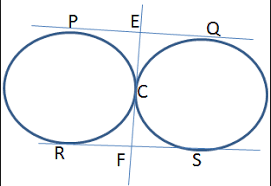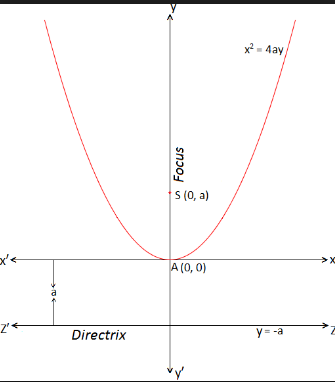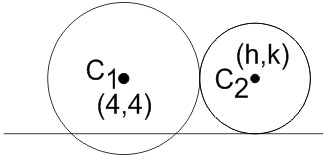#### The centres of those circles which touch the circle, x2+y2−8x−8y−4=0, externally and also touch the x-axis, lie on : Option 1)  a circle.   Option 2) an ellipse which is not a circle.   Option 3)  a hyperbola.   Option 4)  a parabola.As we learnt in

Common tangents of two circles -

When two circles touch  each other externally, there are three common tangents, two of them are direct.

- whereinCircle touching x-axis and having radius r -- wherein

Where f is a variable parameter.

Standard equation of parabola -- whereinCircle:externallywe getOn squaring both sidescompared toRepresent a parabola

Option 1)

a circle.

Incorrect Option

Option 2)

an ellipse which is not a circle.

Incorrect Option

Option 3)

a hyperbola.

Incorrect Option

Option 4)

a parabola.

Correct option

#### divya.saini